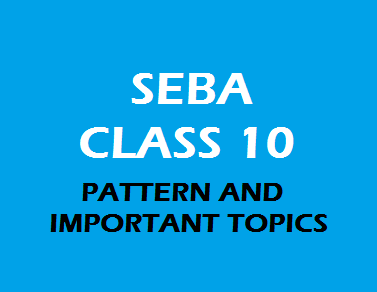# SEBA Class 10: General Mathematics Question Paper Pattern and Syllabus### H.S.L.C (SEBA)GENERAL MATHEMATICS Subject Code – C2

CLASS – X
Theory Total Marks – 90
Interval Assessment –10
General mathematics paper consists of total 15 chapters. Marks allotted to each chapters are stated below:
(सामान्य गणित के पेपर में कुल 15 अध्याय होते हैं। प्रत्येक अध्याय को आवंटित संख्या नीचे बताई गई है:)

 Unit Sub – Unit / Lesson Marks 1.2.3.4.5.6.7.8.9.10.11.12.13.14.15. Real Number Polynomial Pair of Linear equation in two variablesQuadratic equation Arithmetic Progression Triangle Co-ordinate Geometry Introduction to Trigonometry Some application of trigonometry Circle Construction Area related to circles Surface Areas and volumes Statistics Probability 648868995544653 Theory Total Practical 9010 Grand –Total 100
Weightage and Form of Question
This paper is divided into five sections. No. of questions and marks distribution of each section are stated below (यह पेपर पांच खंडों में विभाजित है। प्रत्येक खंड के प्रश्नों और अंकों के वितरण की संख्या नीचे दी गई है)
 Type Marks No. of question 1. Multiple Choice Question (MCQ: Section – A) 1 12 2. Short Answer Types Question  (SA: Section – B) 2 09 3. Short Answer Types Question  (SA: Section – C) 3 10 4. Long Answer Types Question  (LA: Section – D) 4 5 5. Long Answer Types Question (LA: Section – E) 5 10

BLUE PRINT of General Mathematics Paper
Chapter wise marks distribution along with type of questions asked from each chapter are stated below:
***************
(प्रत्येक अध्याय से पूछे गए प्रश्नों के प्रकार के साथ अध्याय वार अंक वितरण नीचे दिए गए हैं:)
 Unit Types of Question MCQSection - AMarks: (1) SASection – BMarks : (2) SASection – CMarks: (3) LASection – DMarks: (4) LASection – EMarks: (5) Total 1. Real Number 1 1 1 6 2. Polynomial 1 1 4 3. Pair of Linear equation of two variable 1 1 1 8 4. Quadratic Equation 1 2 8 5. Arithmetic Progression 1 1 1 6 6. Triangle 1 1 1 8 7. Co-ordinate Geometry 1 1 2 9 8. Introduction to trigonometry 1 4 9 9. Application of Trigonometry 1 1 5 10. Circle 1 1 5 11. Construction 1 4 12. Area related to Circle 1 1 4 13. Surface Area & Volume 1 1 6 14. Statistics 1 5 15. Probability 1 1 3 Total 12 9 10 5 2 100
Syllabus of General Mathematics
Unit – 1: Real Number
Ø  Use Euclid division algorithm to find H.C.F.
Ø  Study about positive odd and even integer’s Form.
Ø  L.C.M. and H.C.F. by Factorization Method.
Ø  Relationship between H.C.F. and L.C.M. of two numbers.
Ø  Method of proving Irrational number.
Ø  Terminating and Non-terminating Decimal Expansion.
Unit – 2: Polynomial
Ø  Relationship between zeroes and coefficient of a polynomial.
Ø  Division algorithm for polynomial.
Unit – 3: Pair of Linear equation in two variables
Ø  Solution of a Pair of linear equation by Graphical Method.
Ø  Solution of a Pair of Linear equation by algebraic Method.
a)      Substitution Method.
b)      Elimination Method.
c)       Cross-multiplication Method.
Ø  Equation Reducible to a Pair of Linear equation in two variables.
Ø  Nature of pair of Linear equation Solution
a)      Unique Solution.
b)      Infinitely Many Solutions.
c)       No Solution.
Ø  Standard form of Quadratic equation.
Ø  Solution of a Quadratic equation by
a)      Factorization.
b)      Completing the Square.
Ø  Nature of roots of Quadratic equation
a)      Two distinct real roots.
b)      Two equal real roots.
c)       No real roots.
Unit – 5: Arithmetic Progression
Ø  Definition & meaning.
Ø  Nth term of an A.P.
Ø  Sum of first nth term of an A.P.
Unit – 6: Triangle
Ø  Meaning & types of triangle.
Ø  Similarity of triangle (Criteria).
Ø  Basic proportionality Theorem and Converse of BPT.
Ø  Area of Similar triangle Theorem.
Ø  Pythagoras Theorem.
Unit – 7: Co-ordinate Geometry
Ø  Distance formula.
Ø  Section formula.
Ø  Mid-point formula.
Ø  Area of triangle.
Unit – 8: Introduction of trigonometry
Ø  Introduction.
Ø  Trigonometry Ratio.
Ø  Trigonometry value of some specific angle.
Ø  Trigonometry ratio of complementary angle.
Ø  Trigonometry Identities.
Unit – 9: Some Application of Trigonometry
Ø  Height and Distance.
Ø  Use Trigonometry Ratio.
Unit – 10: Circle
Ø  Introduction about circle, tangent secant.
Ø  Tangent to a circle.
Ø  Number of tangent from a point on a circle (Theorem).
Unit – 11: Construction
Ø  Division of Line Segment in ratio.
Ø  Construction of Another Triangle in given ratio as compare to existing triangle.
Ø  Construction of tangent to a circle.
Unit – 12: Area related to circle
Ø  Introduction about the area of all 2D shapes.
Ø  Perimeter of 2D shapes.
Ø  Area of sector and segment of a circle.
Ø  Length of sector and segment.
Ø  Area of combined plane figures.
Uni – 13: Surface Area and Volumes
Ø  Surface Area and Volume of –
a)      Cube.
b)      Cuboids.
c)       Cone.
d)      Cylinder.
e)      Sphere.
f)       Hemisphere.
g)      Frustum of a cone.
Ø  Surface Area and Volume of Combination of Solids.
Unit – 14: Statistics
Ø  Mean of Grouped Data.
Ø  Mode of Grouped Data.
Ø  Median of Grouped Data.
Ø  Graphical Representation of Cumulative frequency Distribution.
Unit – 15: Probability
Ø  Introduction.
Ø  Probability of the following:
a)      Coin.
b)      Dice.
c)       Playing Card & another.
Ø  Study about sure event, impossible event.